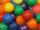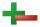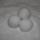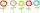Comparing + subtracting - examples

1. Sphere floatingWill float a hollow iron ball with an outer diameter d1 = 20cm and an inside diameter d2 = 19cm in the water? The iron density is 7.8 g/cm 3. (Instructions: Calculate the average sphere density and compare with the density of the water. )
2. RootThe root of the equation ? is: ?
3. BallsI have 19 balls and my friend has 27. About how many has my friend more balls?
4. Opposite numbersCalculate opposite numbers (additive inverse) to given ones:How much and how many times is 72.1 greater than 0.00721?
6. Snow ballsAdam made 26 snowballs. Boris made less snowballs. How many snowballs Boris could do?
7. GardenThe garden grows 312 roses and 389 tulips. Which flowers are more? How many?
8. The HotelThe Holiday Hotel has the same number of rooms on each floor. Rooms are numbered with natural numbers sequentially from the first floor, no number is omitted, and each room has a different number. Three tourists arrived at the hotel. The first one was in r

We apologize, but in this category are not a lot of examples.
Do you have an interesting mathematical example that you can't solve it? Enter it, and we can try to solve it.

To this e-mail address, we will reply solution; solved examples are also published here. Please enter e-mail correctly and check whether you don't have a full mailbox.